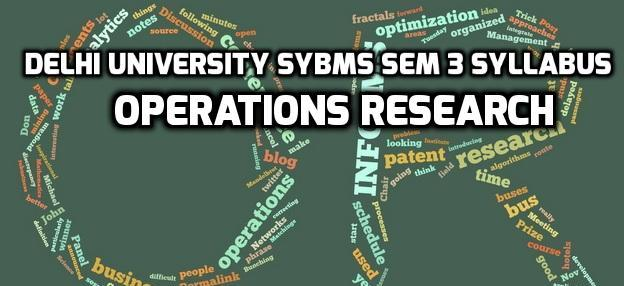# Delhi University SYBMS Sem 3 Syllabus: Operations Research

0Operations Research, a sub-field of mathematics is an application of advanced analytical methods to help make better decisions. Operations Research involves wide range of problem-solving techniques and methods like simulation, mathematical optimization and others which are computational and statistical in nature.

Operations Research (Paper No. 7) is a subject in undergraduate programme of BMS at Delhi University. Operations Research acquaints BMS students with the construction of optimization techniques and models for managerial decision situations. The emphasis is on understanding the concepts formulation and interpretation.

Here we present Delhi University SYBMS Sem 3 Syllabus: Operations Research:

Unit 1 – 18 lectures

1. Linear Programming Formulation, Assumptions and Solution
2. Graphical Solutions
3. Simplex Method
4. Artificial variable techniques (Big M method and Two-phase method)
5. Duality, Sensitivity : Resource and Cost.

Unit 2 – 17 lectures

1. Integer programming: Formulation and solution through Gomorry Cut method
2. Transportation problem – Formulation
3. Initial basic feasible solution by North-West Corner Rule
4. Least Cost Method
5. Vogels Approximation Method (VAM)
6. Transportation Simplex Algorithm
7. Assignment problem – Hungarian method

Unit 3 – 10 lectures

1. Network analysis – Construction of the network diagram
2. Critical path – float and slack analysis
3. Total float
4. Free float
5. Independent float
6. Programme Evaluation Review Technique (PERT)
7. Project Time Crashing

Unit 4 – 5 lectures

1. Game theory – pay off matrix
2. Two person Zero – sum game
3. Pure strategy
5. Dominance Rule
6. Mixed strategy
7. Reduction of m * n game and solution of 2*2, 2*s and 2*2 cases by Graphical and Algebraic methods and formulation to Linear Programming Problem (LPP)

Unit 5 – 6 lectures

1. Decision theory
2. Pay off table
3. Opportunity loss table
4. Expected monetary value
5. Expected opportunity loss
6. Expected value of perfect information and sample information
7. Markov chains – predicting future market shares
8. Equilibrium conditions
9. Limiting probabilities
10. Chapman Kolmogorov equation

Text books and References:

• Operations Research – An introduction, 9th ed. – Hamdy A. Taha
• Operations Research – Theory and Applications, 5th ed – J.K. Sharma
• Operations Research – Kanti Swarup, P.K. Gupta and Man Mohan
• Quantitative Techniques in Management – N.D. Vohra- Tata McGraw Hill

0